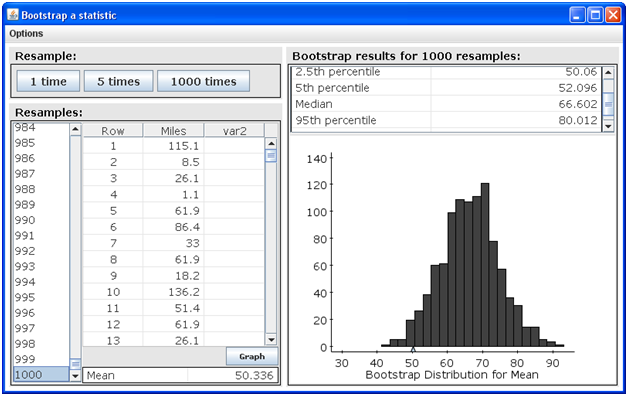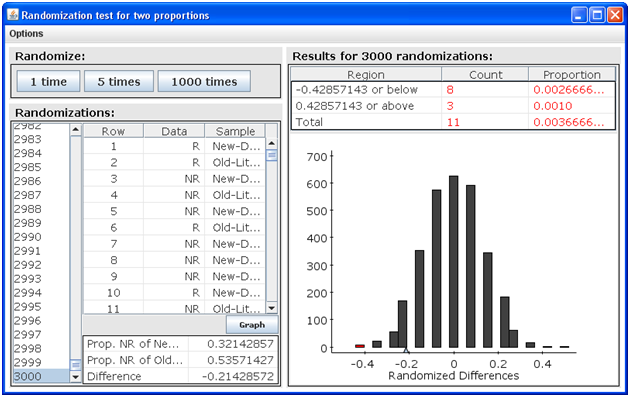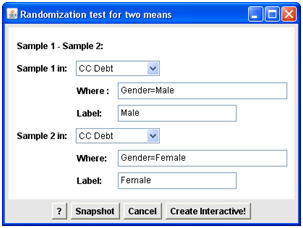## MATH 211 Week 3 Assignment | Embry Riddle Aeronautical University | Assignment Help

MATH 211 Week 3  Assignment  | Embry Riddle Aeronautical University |  Assignment Help

StatCrunch Assignment 2

First save this file to your computer. Answer each of the following questions, then resave the file along with your answers and turn it in using the assignment link in Module 3, Activity 4.The first four problems are worth 10 points each. Problems 5 and 6 are worth 30 points each.

1. If the original sample is 48, 55, 43, 61, 39, which of the following would not be a possible bootstrap sample? Explain why it wouldn’t be.

a) 48, 55, 43, 61, 39

b) 43, 39, 56, 43, 61

c) 55, 48, 55, 48, 61

d) 39, 39, 39, 39, 39

2. The following bootstrap output is for mileage of a random sample of 25 mustang cars.Based on a 90% confidence interval, which of the following would not be a plausible value of the population mean, µ? Explain why it wouldn’t be.

a) 60.01

b) 80.01

c) 55

d) 52

3. Which of the following p-values would provide more evidence in support of the alternate hypothesis and against the null hypothesis? Explain your answer.

a) 0.15

b) 0.85

c) 0.009

d) 0.05

4. In a sample of 56 addicts, 28 were given a new drug to help them overcome their addiction, while the remaining 28 were given the current drug. The following is the output from a randomization test for the difference in the proportion of addicts suffering relapses.

a) What is the difference in proportions in the original data?

b) What is the p-value and what does it mean in terms of this specific problem?In StatCrunch Assignment 1A, you selected a random sample of 30 StatCrunch U students and gathered some survey data regarding those students. Use the StatCrunch data file you created to complete the remainder of this assignment.

5. Construct a bootstrap distribution of the credit card debt data from your sample using 3000 resamples.

a) Paste a copy of your distribution here. (With a PC, you can press the control-alt-fn-F11 keys to copy the window showing the distribution.)

b) What is the mean of your original sample?

c) What is the 95% confidence interval estimate of the population mean obtained from your bootstrap distribution?

6. Suppose you want to compare the amount of credit card debt for males and females. The null hypothesis for this problem would be no difference in the mean amount of credit card debt for males and females and the alternate hypotheses would be that there is a difference in mean amounts of credit card debt.  Conduct a randomization test for two means with 3000 randomizations. (Your input menu should be as follows.)a) Paste the output from your randomization test here.

b) What is the mean difference in credit card debt of the two groups in the original data?

c) What p-value did you get in your randomization? Explain in the context of the problem what the p-value means.

d) Do you think the data support the null hypothesis of no difference in mean credit card debt between males and females or the alternate hypothesis that there is a difference? Explain your answer.